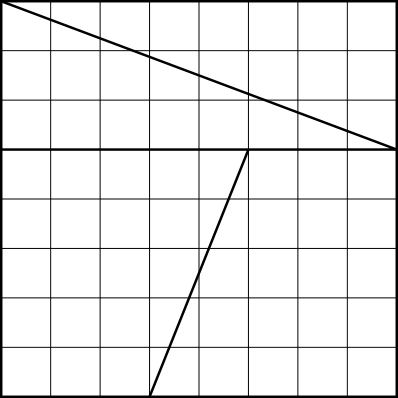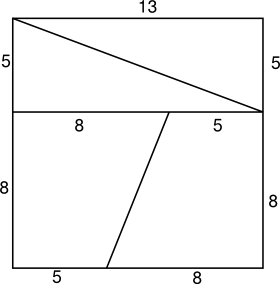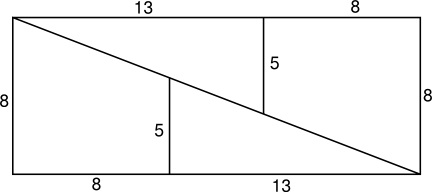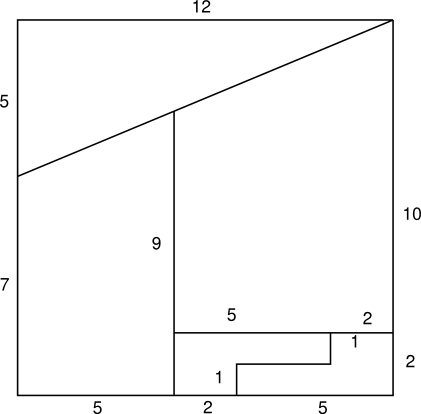#Dissection Puzzles

Math Lair Home > Puzzles & Problems > Dissection Puzzles

There is a class of puzzles that could be termed dissection puzzles, or sometimes dissection paradoxes. These problems involve taking a figure, dissecting it into various parts, and rearranging its parts so that its area, or some other property that should remain the same, apparently changes.

The first example is around 150 years old or so. According to Mathematical Recreations and Essays, it dates back at least as far as 1868. It seems to have gained popularity quite quickly; I've seen it in several newspapers of the 1880s (e.g. the St. Paul Globe, March 21, 1887). Divide an 8×8 square into four pieces as follows:These four pieces can be rearranged into a 5×13 rectangle as shown below. But 5×13 = 65, while 8×8 = 64. So, we've created one unit of area by rearranging some shapes, but that's impossible. So, where did the extra unit of area come from?It may make it easier to see the flaw in the construction if you take a piece of graph paper, cut it up into four pieces, and rearrange it. If you don't see the flaw yet, try that first, and then read the solution.

The solution is as follows: Take a look at the long diagonal of the rectangle. It runs five units down and 13 units to the right. However, the pieces along the long diagonal are two 3×8 triangles and two other shapes made out of 2×5 triangles. 513 is close to 38 and 25, but none of the fractions are equal to one another, so the four pieces do not fit together snugly. If you put the pieces together, you will find that there is a small gap in the middle of the figure, and the gap has an area of exactly 1 unit.

If you're observant, you may have noticed that the key numbers in this dissection (3, 5, 8, and 13) are all Fibonacci numbers. Other Fibonacci numbers can be used to construct a similar dissection. For example the following shape:can be rearanged into:In this case, the original square has an area of 169 square units, while the rectangle has an area of 168 square units, so one square unit was "lost," instead of "gained." Just as there was a small gap 1 square unit in area in the previous dissection, there is a small overlap of 1 square unit in this dissection.

The reason that Fibonacci number appear in this problem is that they have the property that, given three consecutive Fibonacci numbers, the product of the first and third is either 1 larger or 1 smaller than the square of the second. If we take 3, 5, and 8 as an example, 5² = 25, while 3 × 8 = 24. Every Fibonacci number located in an odd position in the Fibonacci series (2, 5, 13, 34, ...) has a square that is 1 more than the product of the two adjacent numbers, while every number located in an even position has a square that is 1 less than that product.

Here's a different dissection puzzle, called the Curry square. This 12×12 square...can apparently be rearranged into a 12×12 square with a 1×1 hole in it:Where did the extra unit go? Again, drawing this figure on graph paper may help. If you do, you'll notice that the diagonal fits flush, so that isn't the problem here. However, you might notice that the side labelled "9" is not exactly 9; actually, it's 9112. Moving this side from the middle to the sides results in the rearranged square having an area of 12×12112, or 145 square units. Subtract the 1-unit "hole," and the area is 144 square units, the same as the original square.

Sources used (see bibliography page for titles corresponding to numbers): 34, 56.